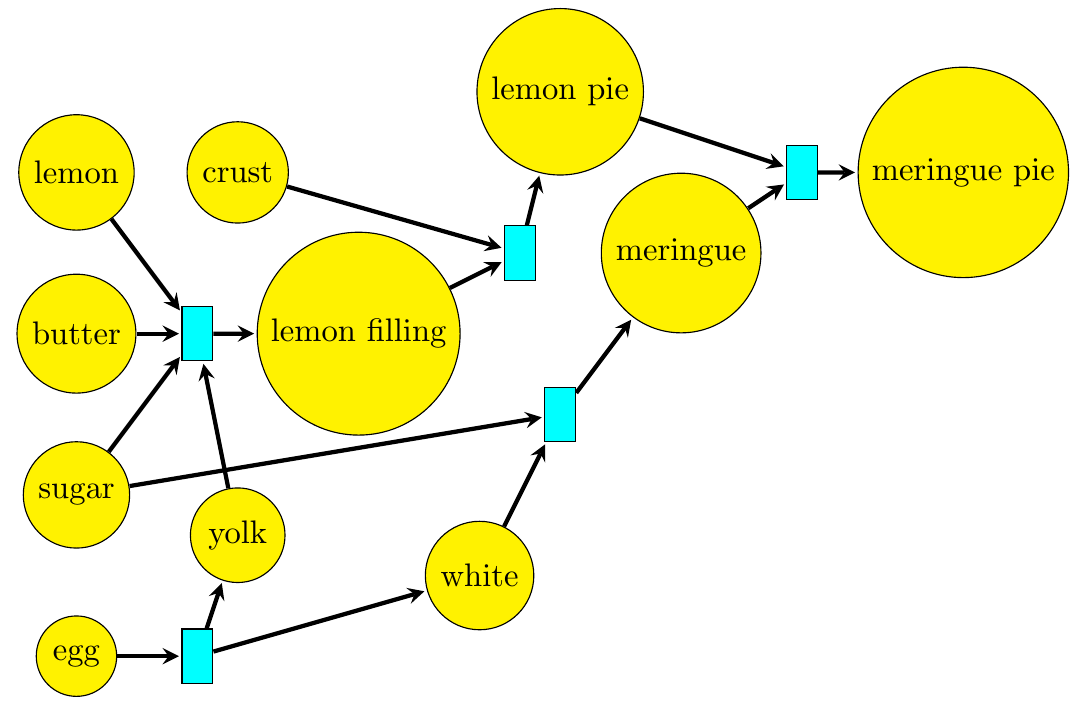Why did I spend the first 33 lectures in a course on category theory talking, not about categories, but about _preorders?_ It's because preorders are categories with at most one morphism from any object \$$x\$$ to any object \$$y\$$. Since there's at most one, we don't need to give it a name: we just write \$$x \le y\$$ if such a morphism exists. And then your experience with inequalities helps you understand what's going on! But beware! Since there's at at most one morphism from any object \$$x\$$ to any object \$$y\$$ in a preorder, any time you have two morphisms \$$f, g : x \to y\$$ they are automatically equal. This hid one of the most important features of category theory, namely _equations between morphisms_. The definition of category theory starts out with three fundamental equations between morphisms: the associative law $(h \circ g) \circ f = h \circ (g \circ f)$ whenever we have three morphisms that can be composed, and the left and right unit laws $1_y \circ f = f = f \circ 1_x$ whenever we have a morphism \$$f : x \to y\$$. These were all _automatic_ and thus _not mentioned_ in our study of preorders. As we continue, we'll see a lot more equations between morphisms - so get ready for this change of focus! Eventually, we'll take almost everything we've done with preorders and generalize it to categories. Here's a little list of these things. It won't make sense yet, so don't worry about it too much - but I still want to show it to you: * Preorders are a special case of ["categories"](https://en.wikipedia.org/wiki/Category_theory): they are categories with at most one object from any object to any object. * Monotone maps between preorders are a special case of ["functors"](https://en.wikipedia.org/wiki/Functor) between categories: they are functors between categories that happen to be preorders. * Left and right adjoint monotone maps between preorders are a special case of ["left and right adjoint functors"](https://en.wikipedia.org/wiki/Adjoint_functors) between categories: they are left and right functors between categories that happen to be preorders. * Monoidal preorders are a special case of ["monoidal categories"](https://en.wikipedia.org/wiki/Monoidal_category): they are monoidal categories that happen to be preorders. * Symmetric monoidal preorders are a special case of ["symmetric monoidal categories"](https://en.wikipedia.org/wiki/Symmetric_monoidal_category): they are symmetric monoidal categories that happen to be preorders. * Categories enriched in monoidal preorders are a special case of ["categories enriched in monoidal categories"](https://en.wikipedia.org/wiki/Enriched_category): they are categories enriched in monoidal categories that happen to be preorders. So, there's a lot of stuff to learn - but whenever you meet one of these concepts and need some examples and insights, you can fall back on your knowledge of preorders! In [Lecture 25](https://forum.azimuthproject.org/discussion/2090/lecture-25-chapter-2-reaction-networks/p1) we saw this Petri net:Since we were studying preorders we treated each reaction, like "fill crust", as a mere _inequality_. The _name_ of the reaction didn't really matter in our work. If there were two different ways to transform crust and filling into a lemon pie, that wouldn't affect anything at all. Now that we're studying categories we can treat each reaction as a _morphism_, and write: $[\textrm{fill crust}] : [\textrm{crust}] + [\textrm{filling}] \to [\textrm{lemon pie}] .$ This lets us distinguish between two different morphisms from \$$[\textrm{crust}] + [\textrm{filling}]\$$ to \$$[\textrm{lemon pie}] \$$. For example, we can distinguish between $[\textrm{fill crust slowly}] : [\textrm{crust}] + [\textrm{filling}] \to [\textrm{lemon pie}] .$ and $[\textrm{fill crust quickly}] : [\textrm{crust}] + [\textrm{filling}] \to [\textrm{lemon pie}]$ This is very important when we're studying real-world manufacturing processes, where there are often several ways to turn the same inputs into the same outputs. You'll notice the \$$+\$$ sign in these formulas, which lets us combine resources. That's a sign that we're working with a _symmetric monoidal_ preorder, or symmetric monoidal category. That's too fancy for us now: we'll start by studying plain old categories. So, instead of using _manufacturing_ as an example, we'll look at something else: _databases_. **Puzzle 102.** What is the category with the fewest morphisms that is not a preorder? **Puzzle 103.** Figure out the best way to take any category \$$\mathcal{C}\$$ and "squash it down", getting a preorder whose elements are the objects of \$$\mathcal{C}\$$. **[To read other lectures go here.](http://www.azimuthproject.org/azimuth/show/Applied+Category+Theory#Chapter_3)**